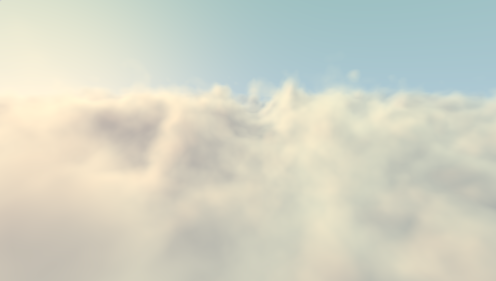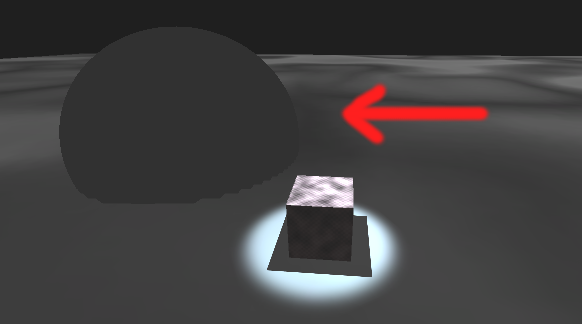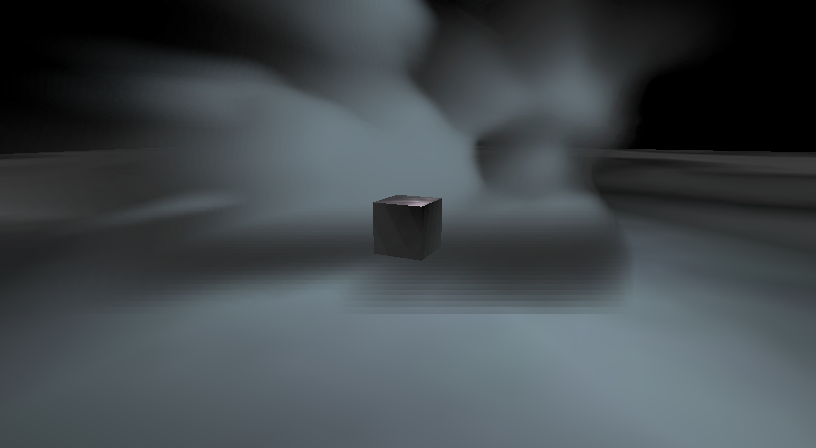## So what are volumetrics?

Basically, things like clouds.You may have seen the amazing visualization 'Clouds' on shadertoy, and wondered how on earth it works.

```#define MARCH(STEPS,MAPLOD) for(int i=0; i2.0 || sum.a > 0.99 ) break; float den = MAPLOD( pos ); if( den>0.01 ) { float dif =  clamp((den - MAPLOD(pos+0.3*sundir))/0.6, 0.0, 1.0 ); sum = integrate( sum, dif, den, bgcol, t ); } t += max(0.1,0.02*t); }

vec4 raymarch( in vec3 ro, in vec3 rd, in vec3 bgcol ) {
vec4 sum = vec4(0.0);
float t = 0.0;
MARCH(30,map5);
MARCH(30,map4);
MARCH(30,map3);
MARCH(30,map2);
return clamp( sum, 0.0, 1.0 );
}
```

Yeah?

Well. That's a bit too complicated for me, but in principal what this is doing is defining a volume in space, procedurally, and then 'raymarching' from each pixel on the canvas out into the procedural volume and accumulating lighting and volume data at each step. Kind of like simplified raytracing.

In practice though, `#define MARCH` is a pretty complicated function.

Let's start much smaller.

# A procedural sphere.

You can define a sphere as a point and a radius.

Any other point is either inside or outside the sphere based on the simple equation:

```bool inside(point) { return distance(center, point) < radius; }
```

So on a completely simple scale, to render this object using a shader all we need to do is check the world coordinate of each fragment, and return transparent for 'not in sphere' and white for 'in sphere'.(Yeah yeah, it's not white. That's the fragment color, after lighting its grey...)

The key part of this shader is:

```    // See if a value is bounded inside 'magic volume'
// In this case; a sphere.
bool bounded(float3 a) {
return distance(a, _center) < _size;
}

// Calculate the relative distance from the center point
// Notice unity helpfully provides an IN struct with worldPos.
void surf (Input IN, inout SurfaceOutput o) {
o.Alpha = 0;
o.Albedo = fixed3(0, 0, 0);
if (bounded(IN.worldPos)) {
o.Albedo = fixed3(1, 1, 1);
o.Alpha = 1;
}
}
```

Now, you may say, surely this shader is only describing a single 'slice' through the volume, not the entire thing, why is it a sphere, not a circle?

Quite right. I'm cheating; in this case the mesh is a series of parallel quads spaced close to each other, describing the volume as a series of slices. This is the alternative to raymarching for displaying volumes.

Notice the 'stepped' edges of the sphere; the more slices you render, the slower it is to render; but it looks better.

The downside to raymarching is that although the geometry is simple, objects are either occluded or not; there is not 'partial' entry into the rendering volume. Ultimately a combination of the two is the best solution, but we'll leave that for another day.

# Clouds?

Anyway, on to clouds.

Lets have a look at a more complex example:Check out the live demo (28MB webgl)

Although it looks more complicated, the example here is basically the same as the sphere volume renderer:

```// Calculate edge size
float edge(float3 a) {
return calc_offset_xy(a) + _size * calc_offset_zx(a);
}

// Distance between two points
float distance(float3 a, float3 b) {
float x = (a - b) * (a - b);
float y = (a - b) * (a - b);
float z = (a - b) * (a - b);
return sqrt(x + y + z);
}

// Calculate the edge distance as a fractional value
float edge_fraction(float3 a) {
return 1.0 - distance(a, _center) / edge(a);
}

// Calculate the relative distance from the center point
void surf (Input IN, inout SurfaceOutput o) {
float alpha = edge_fraction(IN.worldPos);
o.Albedo = fixed3(0, 0, 0);
o.Alpha = 0;
if (alpha > 0) {
o.Albedo = float3(alpha, alpha, alpha);
o.Alpha = alpha * 0.5;
}
}
```

The alpha is based on the distance to the center of the sphere, and the only difference is that a texture map is used to generate smooth looking interesting curves in `calc_offset_xy`.

The animation is generated by using a sin curve to move the sample point on the noise texture over time:

```float time() {
return _seed + _Time / 8;
}

float calc_offset_xy(float3 a) {
return tex2D(
_xy,
float2(
sin(time() + a / _size),
sin(time() + a / _size))
);
}
```

Notice particularly that because this is a surface shader, lighting is automatically applied to the slices, giving it convincing (fake) volumetric illumination.

I'm still getting my head around how exactly the best way to render these volumes is; but for now, the results are quite promising.

The full code for the shader and volume rendering helper are:

```Shader "Shaders/Volume/BasicFogVolumeMaterial" {
Properties {
_xy ("XY", 2D) = "white" {}
_center ("Center", Vector) = (0, 0, 0, 0)
_size ("Size", Float) = 0
_seed ("Seed", Float) = 0
}
Blend SrcAlpha OneMinusSrcAlpha
Tags { "Queue" = "Transparent" "RenderType"="Transparent" }
CGPROGRAM

#pragma surface surf Lambert alpha

uniform float3 _center;
uniform float _size;
uniform float _seed;

sampler2D _xy;

struct Input {
float3 worldPos;
};

float time() {
return _seed + _Time / 8;
}

float calc_offset_xy(float3 a) {
return tex2D(
_xy,
float2(
sin(time() + a / _size),
sin(time() + a / _size))
);
}

float calc_offset_yz(float3 a) {
return tex2D(
_xy,
float2(
sin(time() + a / _size),
sin(time() + a / _size))
);
}

float calc_offset_zx(float3 a) {
return tex2D(
_xy,
float2(
sin(time() + a / _size),
sin(time() + a / _size))
);
}

// Calculate edge size
float edge(float3 a) {
return calc_offset_xy(a) + _size * calc_offset_zx(a);
}

// Distance between two points
float distance(float3 a, float3 b) {
float x = (a - b) * (a - b);
float y = (a - b) * (a - b);
float z = (a - b) * (a - b);
return sqrt(x + y + z);
}

// Calculate the edge distance as a fractional value
float edge_fraction(float3 a) {
return 1.0 - distance(a, _center) / edge(a);
}

// Calculate the relative distance from the center point
void surf (Input IN, inout SurfaceOutput o) {
float alpha = edge_fraction(IN.worldPos);
o.Albedo = fixed3(0, 0, 0);
o.Alpha = 0;
if (alpha > 0) {
o.Albedo = float3(alpha, alpha, alpha);
o.Alpha = alpha * 0.5;
}
}

ENDCG
}
Fallback "Diffuse"
}
```
```using System;
using System.Collections.Generic;
using UnityEngine;

/// Generate a series of parallel planes relative to the camera
[RequireComponent(typeof(MeshFilter))]
[RequireComponent(typeof(MeshRenderer))]
public class SimpleVolumeRenderer : MonoBehaviour {

[Tooltip("The camera to make this volume viewable from")]
public new UnityEngine.Camera camera;

[Tooltip("The size of the frame to generate for this object")]
public float size = 1f;

[Tooltip("The number of slices to mesh")]
public int slices = 10;
private int slices_;

[Tooltip("The slice interval")]
public float sliceGap = 0.1f;

/// Internal vertex buffer
private Vector3[] points;

/// Internal center id name
private int centerId;
private Renderer renders;

public void Start() {
Build();
renders = GetComponent();
renders.material.SetFloat("Seed", UnityEngine.Random.Range(0.0f, 5.0f));
}

public void Update() {
if (slices_ != slices) {
Build();
}
Rebuild();
renders.material.SetVector(centerId, this.transform.position);
}

/// Generate a quad facing the camera at the given offset
private void BuildQuad(Vector3 origin, Vector3 up, Vector3 right, int offset) {
points[offset * 4 + 0] = origin + right - up;
points[offset * 4 + 1] = origin + right + up;
points[offset * 4 + 2] = origin - right + up;
points[offset * 4 + 3] = origin - right - up;
}

/// Generate new points for each quad
public Vector3[] MeshPoints() {
if ((points == null) || (points.Length != 4 * slices)) {
points = new Vector3[4 * slices];
}
var offset = size / 2f;
var normal = (-1f * camera.transform.forward).normalized;
var up = camera.transform.up.normalized * offset;
var right = Vector3.Cross(normal, up).normalized * offset;
for (var i = 0; i < slices; ++i) {
var o = i - slices / 2;
var src = - sliceGap * o * normal;
}
return points;
}

/// Rebuild vertex points only
public void Rebuild() {
MeshFilter meshFilter = gameObject.GetComponent();
meshFilter.mesh.vertices = MeshPoints();
}

/// Build all details of the mesh
public void Build() {
if (camera == null) {
return;
}

MeshFilter meshFilter = gameObject.GetComponent();

// Generate a set of meshes
var mesh = new Mesh();
mesh.Clear();

var verts = MeshPoints();
mesh.vertices = verts;

var uvs = new Vector2[4 * slices];
for (var i = 0; i < slices; ++i) {
uvs[i * 4 + 0]  = new Vector2(0f, 0f);
uvs[i * 4 + 1]  = new Vector2(1f, 0f);
uvs[i * 4 + 2]  = new Vector2(1f, 1f);
uvs[i * 4 + 3]  = new Vector2(0f, 1f);
}
mesh.uv = uvs;

// Always aim at camera
var normal = (-1f * camera.transform.forward).normalized;
var normals = new Vector3[4 * slices];
for (var i = 0; i < slices; ++i) {
normals[i * 4 + 0] = normal;
normals[i * 4 + 1] = normal;
normals[i * 4 + 2] = normal;
normals[i * 4 + 3] = normal;
}
//mesh.normals = normals;

var triangles = new int[6 * slices];
for (var i = 0; i < slices; ++i) {
triangles[i * 6 + 0] = i * 4 + 2;
triangles[i * 6 + 1] = i * 4 + 1;
triangles[i * 6 + 2] = i * 4 + 0;
triangles[i * 6 + 3] = i * 4 + 0;
triangles[i * 6 + 4] = i * 4 + 3;
triangles[i * 6 + 5] = i * 4 + 2;
}
mesh.triangles = triangles;

mesh.RecalculateBounds();
mesh.RecalculateNormals();
mesh.Optimize();
meshFilter.mesh = mesh;
slices_ = slices;
}

public void OnDrawGizmos() {
Gizmos.color = new Color(0.5f, 0.5f, 1.0f, 0.75f);
Gizmos.DrawCube(transform.position, transform.lossyScale);
}
}
}
```